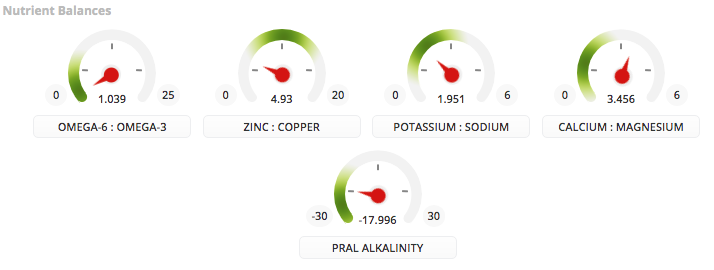Nutrient balancesI know this subject has been discussed previously but I cannot seem to find a concrete example of how those nutrient balances work, what's the exact calculation?
So could someone tell me how to calculate my Omega 6 : Omega 3 ratio with those numbers in the picture below for example?Thanks•Hi there mrgndbtn,

Your ratio for each of these pairs of nutrients is shown in the centre of each nutrient balance. You can calculate each number by finding the values for each individual nutrient in your targets and dividing them.

For example, your omega-6 intake divided by your omega-3 intake = 1.039

Karen Stark
cronometer.com
As always, any and all postings here are covered by our T&Cs:
https://forums.cronometer.com/discussion/27/governing-terms-and-disclaimer

•Hi Karen,
Just one thing, you know how the ratios are normally written like so: 4:1 for example.
How would that translate with my result 1.039?
Knowing that omega 6 = 6.1 and omega 3 = 5.9?

•omega 6 : omega 3
6.1 : 5.9
6.1/5.9 = 1.039

•Ok so the way it's displayed with the little gauge showing 0 - (some number) and then a green area (optimal range?) and then the dial that points to an unknown area seems to me, not useful at all. What rage or number is the green optimal area on, and why is it a decimal and not a ratio displayed under this dial? How is this useful if I have to go to the nutrition details and look up both values to figure out how the decimal value was calculated 6-3 or 3-6? Once you have a decimal calculated what good does that do you anyway? Any research you do compares such micro nutrients via a ratio. Honestly, I've been racking my brain trying to figure how any of these nutrient balance dials are useful, and I can't seem to figure it out. It just seems a bit gimmicky to me at the moment. Sorry. Although, I was very happy to initially see these dials as they could be extremely useful. I might recommend that it show the actual ratio under the dial and at least a number that would be optimal even in decimal form for the green area as long as you knew if 1.039 was 6-3 or 3-6, right? This would easily turn such a tool from useless into something very helpful.

You know, it doesn't even need a decimal value at all, If it looked something like the following for example:

Omega-6 : Omega-3
(Actual Ratio)
12.5 : 1

(Normal Ratio) (Optimal Range)
3 : 1 1:1 - 3:1

This is essentially the information attempted to be conveyed by these dials right?

•lol That didn't display the way I typed it but oh well you get the idea.